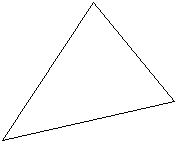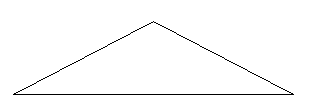# Try to draw rough sketches of            (a) a scalene acute angled triangle.            (b) an obtuse angled isosceles triangle.

H Harsh Kankaria

(a) a scalene acute angled triangle. :

Scalene : All side of different length

Acute angled : All angles less than(b) an obtuse angled isosceles triangle

Isosceles traingle: Only two sides are of equal length

Obtuse angled : At least one angle greater thanExams
Articles
Questions